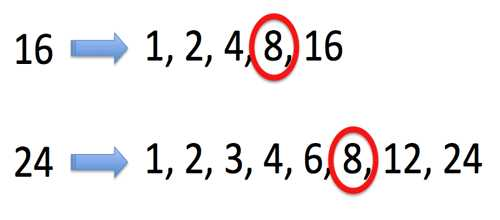Greatest Common Factor

The Greatest Common Factor (GCF) is the largest number that is a common factor of two or more numbers.

The GCF of two numbers is the greatest number that is a factor of both of the numbers. Take the numbers 50 and 30.

50 = 10 • 5

30 = 10 • 3

Their greatest common factor is 10 since 10 is the greatest factor that both numbers have in common.

How to find the greatest common factor:

• Determine if there is a common factor of the numbers. A common factor is a number that will divide into both numbers evenly. Two is a common factor of 4 and 14.
• Divide all of the numbers by this common factor.
• Repeat this process with the resulting numbers until there are no more common factors.
• Multiply all of the common factors together to find the Greatest Common Factor.Explanation with example

It’s often useful in solving math problems to be able to find the largest factor that divides two numbers. We call this the greatest common factor, or GCF. Let’s find the GCF of 30 and 45. First, we find the prime factors of each number, using prime factorization.

30 = 2 × 3 × 5

45 = 3 × 3 × 5

Next, identify those prime factors that both numbers have in common, and multiply them. Here, both 3 and 5 are common factors. The GCF is 3 times 5, or 15.

3 × 5 = 15 ← GCF

Examples,

Find the GCF of these pairs of numbers.

(14, 49)

Solution: List the prime factors of each.

14 = 2 × 7

49 = 7 × 7

7 is the only common factor; therefore, 7 is the GCF.

(15, 75)

Solution: List the prime factors of each.

15 = 3 × 5

75 = 3 × 5 × 5

3 and 5 are common; therefore, 3 × 5 = 15 is the GCF.

Information Source: